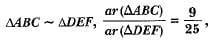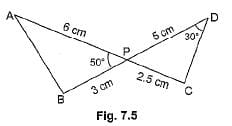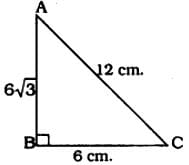Practice Test: Triangles

# Practice Test: Triangles - Class 10

Test Description

## 10 Questions MCQ Test Mathematics (Maths) Class 10 - Practice Test: Triangles

Practice Test: Triangles for Class 10 2023 is part of Mathematics (Maths) Class 10 preparation. The Practice Test: Triangles questions and answers have been prepared according to the Class 10 exam syllabus.The Practice Test: Triangles MCQs are made for Class 10 2023 Exam. Find important definitions, questions, notes, meanings, examples, exercises, MCQs and online tests for Practice Test: Triangles below.
Solutions of Practice Test: Triangles questions in English are available as part of our Mathematics (Maths) Class 10 for Class 10 & Practice Test: Triangles solutions in Hindi for Mathematics (Maths) Class 10 course. Download more important topics, notes, lectures and mock test series for Class 10 Exam by signing up for free. Attempt Practice Test: Triangles | 10 questions in 10 minutes | Mock test for Class 10 preparation | Free important questions MCQ to study Mathematics (Maths) Class 10 for Class 10 Exam | Download free PDF with solutions
 1 Crore+ students have signed up on EduRev. Have you?
Practice Test: Triangles - Question 1

### The length of each side of a rhombus whose diagonals are of lengths 10 cm and 24 cm is

Practice Test: Triangles - Question 2

### Sides of triangles are (i) 3 cm, 4 cm, 6 cm. (ii) 4 cm, 5 cm, 6 cm. (iii) 7 cm, 24 cm, 25 cm (iv) 5 cm, 12 cm, 14 cm. Which of these is right triangle?

Detailed Solution for Practice Test: Triangles - Question 2

On verification, triangle with sides 7 cm, 24 cm and 25 cm is a right triangle
∵ (25)2 = (7)2 + (24)2.

Practice Test: Triangles - Question 3

### IfBC = 21 cm, then EF is equal to

Practice Test: Triangles - Question 4

A square and a rhombus are always

Practice Test: Triangles - Question 5

It is given that ΔABC ~ ΔDFE, ∠A = 30°, ∠C = 50°, AB = 5 cm, AC = 8 cm and DF = 7.5 cm. Then, the following is true:

Practice Test: Triangles - Question 6

In Fig. 7.5, two line segments AC and BD intersect each other at the point P such that PA = 6 cm, PB = 3 cm, PC = 2.5 cm, PD = 5 cm, ∠APB = 50° and ∠CDP = 30°. Then, ∠PBA is equal toPractice Test: Triangles - Question 7

In ΔLMN and ΔPQR, ∠L = ∠P, ∠N = ∠R and MN = 2QR. Then the two triangles are

Practice Test: Triangles - Question 8

If in triangles ABC and DEF, AB/EF = AC/DE, then they will be similar when

Practice Test: Triangles - Question 9

It is given that ar(ΔABC) = 81 square units and ar(ΔDEF) = 64 square units. If ΔABC ~ ΔDEF, then

Practice Test: Triangles - Question 10

In triangle ABC, if AB = 6√3 cm, AC = 12 cm and BC = 6 cm, then ∠B is

Detailed Solution for Practice Test: Triangles - Question 10Here largest side is 12 cm.
If the square of the hypotenuse is equal to the square of the other two sides, then it is a right-angled triangle.

∴ c2 = a2 + b2

AC2 = AB2 + BC2

(12)2 = (63)2 + (6)2

44 = 36 × 3 + 36

144 = 108 + 36

144 = 144

∴ ∆ABC is a right angled triangle and angle opposite to hypotenuse, ∠B = 90°.

## Mathematics (Maths) Class 10

115 videos|478 docs|129 tests
Information about Practice Test: Triangles Page
In this test you can find the Exam questions for Practice Test: Triangles solved & explained in the simplest way possible. Besides giving Questions and answers for Practice Test: Triangles, EduRev gives you an ample number of Online tests for practice

## Mathematics (Maths) Class 10

115 videos|478 docs|129 tests